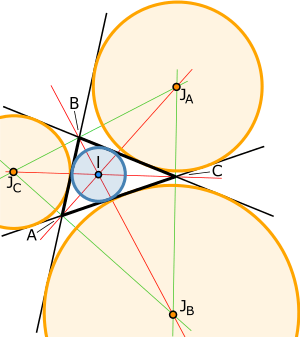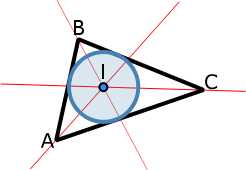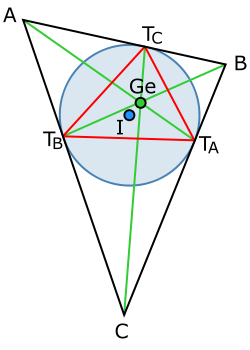# Incircle and excircles of a triangle

"Incircle" redirects here. For incircles of non-triangle polygons, see Tangential quadrilateral and Tangential polygon.A triangle (black) with incircle (blue), incenter (I), excircles (orange), excenters (JA,JB,JC), internal angle bisectors (red) and external angle bisectors (green). The green triangle is the excentral triangle.

In geometry, the incircle or inscribed circle of a triangle is the largest circle contained in the triangle; it touches (is tangent to) the three sides. The center of the incircle is a triangle center called the triangle's incenter.

An excircle or escribed circle of the triangle is a circle lying outside the triangle, tangent to one of its sides and tangent to the extensions of the other two. Every triangle has three distinct excircles, each tangent to one of the triangle's sides.

The center of the incircle, called the incenter, can be found as the intersection of the three internal angle bisectors. The center of an excircle is the intersection of the internal bisector of one angle (at vertex A, for example) and the external bisectors of the other two. The center of this excircle is called the excenter relative to the vertex A, or the excenter of A. Because the internal bisector of an angle is perpendicular to its external bisector, it follows that the center of the incircle together with the three excircle centers form an orthocentric system.:p. 182

Polygons with more than three sides do not all have an incircle tangent to all sides; those that do are called tangential polygons. See also Tangent lines to circles.

## Relation to area of the triangle

The radii of the incircles and excircles are closely related to the area of the triangle.

### IncircleSupposehas an incircle with radius r and center I. Let a be the length of BC, b the length of AC, and c the length of AB. Now, the incircle is tangent to AB at some point C′, and sois right. Thus the radius C'I is an altitude of. Therefore,has base length c and height r, and so has area. Similarly,has areaandhas area. Since these three triangles decompose, we see thatandwhereis the area ofandis its semiperimeter.

For an alternative formula, consider. This is a right-angled triangle with one side equal to r and the other side equal to. The same is true for. The large triangle is composed of 6 such triangles and the total area is:### Excircles

The radii of the excircles are called the exradii. Let the excircle at side AB touch at side AC extended at G, and let this excircle's radius beand its center be. Thenis an altitude of, sohas area. By a similar argument,has areaandhas area. Thus.

So, by symmetry,.

By the Law of Cosines, we haveCombining this with the identity, we haveBut, and sowhich is Heron's formula.

Combining this with, we haveSimilarly,givesandFrom these formulas one can see that the excircles are always larger than the incircle and that the largest excircle is the one tangent to the longest side and the smallest excircle is tangent to the shortest side. Further, combining these formulas yields:The ratio of the area of the incircle to the area of the triangle is less than or equal to, with equality holding only for equilateral triangles.

## Incenter and excenters

A triangle's incenter (the center of its incircle) has trilinear coordinatesIts excenters (the centers of its excircles) have trilinears## Related constructions

### Nine-point circle and Feuerbach point

The circle tangent to all three of the excircles as well as the incircle is known as the nine-point circle. The point where the nine-point circle touches the incircle is known as the Feuerbach point.

### Gergonne triangle and pointThe Gergonne triangle (of ABC) is defined by the 3 touchpoints of the incircle on the 3 sides. The touchpoint opposite A is denoted TA, etc.

This Gergonne triangle TATBTC is also known as the contact triangle or intouch triangle of ABC. Its area iswhere,,are the area, radius of the incircle and semiperimeter of the original triangle, and,,are the side lengths of the original triangle. This is the same area as that of the extouch triangle.

The three lines ATA, BTB and CTC intersect in a single point called the Gergonne point, denoted as Ge - X(7). The Gergonne point lies in the open orthocentroidal disk punctured at its own center, and could be any point therein.

Interestingly, the Gergonne point of a triangle is the symmedian point of the Gergonne triangle. For a full set of properties of the Gergonne point see.

Trilinear coordinates for the vertices of the intouch triangle are given by

•••Trilinear coordinates for the Gergonne point are given by,

or, equivalently, by the Law of Sines,.

### Nagel triangle and point

The Nagel triangle of ABC is denoted by the vertices XA, XB and XC that are the three points where the excircles touch the reference triangle ABC and where XA is opposite of A, etc. This triangle XAXBXC is also known as the extouch triangle of ABC. The circumcircle of the extouch triangle XAXBXC is called the Mandart circle. The three lines AXA, BXB and CXC are called the splitters of the triangle; they each bisect the perimeter of the triangle, and they intersect in a single point, the triangle's Nagel point Na - X(8).

Trilinear coordinates for the vertices of the extouch triangle are given by

•••Trilinear coordinates for the Nagel point are given by,

or, equivalently, by the Law of Sines,.

It is the isotomic conjugate of the Gergonne point.

### Incentral and excentral triangles

The points of intersection of the interior angle bisectors of ABC with the segments BC, CA, AB are the vertices of the incentral triangle. Trilinear coordinates for the vertices of the incentral triangle are given by

•••The excentral triangle of a reference triangle has vertices at the centers of the reference triangle's excircles. Its sides are on the external angle bisectors of the reference triangle (see figure at top of page). Trilinear coordinates for the vertices of the excentral triangle are given by

•••## Equations for four circles

Let x : y : z be a variable point in trilinear coordinates, and let u = cos2(A/2), v = cos2(B/2), w = cos2(C/2). The four circles described above are given equivalently by either of the two given equations::p. 210–215

• Incircle:• A-excircle:• B-excircle:• C-excircle:## Euler's theorem

Euler's theorem states that in a triangle:where R and rin are the circumradius and inradius respectively, and d is the distance between the circumcenter and the incenter.

For excircles the equation is similar:where rex is the radius of one of the excircles, and d is the distance between the circumcenter and this excircle's center.   

## Other incircle properties

Suppose the tangency points of the incircle divide the sides into lengths of x and y, y and z, and z and x. Then the incircle has the radiusand the area of the triangle isIf the altitudes from sides of lengths a, b, and c are ha, hb, and hc then the inradius r is one-third of the harmonic mean of these altitudes, i.e.The product of the incircle radius r and the circumcircle radius R of a triangle with sides a, b, and c is:p. 189, #298(d)Any line through a triangle that splits both the triangle's area and its perimeter in half goes through the triangle's incenter (the center of its incircle). There are either one, two, or three of these for any given triangle.

Denoting the center of the incircle of triangle ABC as I, we haveand:p.121,#84The distance from any vertex to the incircle tangency on either adjacent side is half the sum of the vertex's adjacent sides minus half the opposite side. Thus for example for vertex B and adjacent tangencies TA and TC,The incircle radius is no greater than one-ninth the sum of the altitudes.:p. 289

The squared distance from the incenter I to the circumcenter O is given by:p.232and the distance from the incenter to the center N of the nine point circle is:p.232The incenter lies in the medial triangle (whose vertices are the midpoints of the sides).:p.233, Lemma 1

## Other excircle properties

The circular hull of the excircles is internally tangent to each of the excircles, and thus is an Apollonius circle. The radius of this Apollonius circle iswhere r is the incircle radius and s is the semiperimeter of the triangle.

The following relations hold among the inradius r, the circumradius R, the semiperimeter s, and the excircle radii ra, rb, rc:The circle through the centers of the three excircles has radius 2R.

If H is the orthocenter of triangle ABC, then## Generalization to other polygons

Some (but not all) quadrilaterals have an incircle. These are called tangential quadrilaterals. Among their many properties perhaps the most important is that their two pairs of opposite sides have equal sums. This is called the Pitot theorem.

More generally, a polygon with any number of sides that has an inscribed circleone that is tangent to each sideis called a tangential polygon.

## Notes

1. Kay (1969, p. 140)
2. Altshiller-Court (1925, p. 74)
3. Altshiller-Court (1925, p. 73)
4. Kay (1969, p. 117)
5. Johnson, Roger A., Advanced Euclidean Geometry, Dover, 2007 (orig. 1929).
6. Coxeter, H.S.M. "Introduction to Geometry 2nd ed. Wiley, 1961.
7. Altshiller-Court (1925, p. 79)
8. Baker, Marcus, "A collection of formulae for the area of a plane triangle," Annals of Mathematics, part 1 in vol. 1(6), January 1885, 134-138. (See also part 2 in vol. 2(1), September 1885, 11-18.)
9. Minda, D., and Phelps, S., "Triangles, ellipses, and cubic polynomials", American Mathematical Monthly 115, October 2008, 679-689: Theorem 4.1.
10. Weisstein, Eric W. "Contact Triangle." From MathWorld--A Wolfram Web Resource. http://mathworld.wolfram.com/ContactTriangle.html
11. Christopher J. Bradley and Geoff C. Smith, "The locations of triangle centers", Forum Geometricorum 6 (2006), 57--70. http://forumgeom.fau.edu/FG2006volume6/FG200607index.html
12. Dekov, Deko (2009). "Computer-generated Mathematics : The Gergonne Point" (PDF). Journal of Computer-generated Euclidean Geometry. 1: 114.
13. Whitworth, William Allen. Trilinear Coordinates and Other Methods of Modern Analytical Geometry of Two Dimensions, Forgotten Books, 2012 (orig. Deighton, Bell, and Co., 1866). http://www.forgottenbooks.com/search?q=Trilinear+coordinates&t=books
14. Nelson, Roger, "Euler's triangle inequality via proof without words," Mathematics Magazine 81(1), February 2008, 58-61.
15. Johnson, R. A. Modern Geometry, Houghton Mifflin, Boston, 1929: p. 187.
16. Emelyanov, Lev, and Emelyanova, Tatiana. "Euler’s formula and Poncelet’s porism", Forum Geometricorum 1, 2001: pp. 137–140.
17. Chu, Thomas, The Pentagon, Spring 2005, p. 45, problem 584.
18. Bell, Amy, "Hansen’s right triangle theorem, its converse and a generalization", Forum Geometricorum 6, 2006, 335–342.
19. Kodokostas, Dimitrios, "Triangle Equalizers," Mathematics Magazine 83, April 2010, pp. 141-146.
20. Allaire, Patricia R.; Zhou, Junmin; and Yao, Haishen, "Proving a nineteenth century ellipse identity", Mathematical Gazette 96, March 2012, 161-165.
21. Altshiller-Court, Nathan. College Geometry, Dover Publications, 1980.
22. Mathematical Gazette, July 2003, 323-324.
23. Posamentier, Alfred S., and Lehmann, Ingmar. The Secrets of Triangles, Prometheus Books, 2012.
24. Franzsen, William N. (2011). "The distance from the incenter to the Euler line" (PDF). Forum Geometricorum. 11: 231–236. MR 2877263..
25. Grinberg, Darij, and Yiu, Paul, "The Apollonius Circle as a Tucker Circle", Forum Geometricorum 2, 2002: pp. 175-182.
26. Stevanovi´c, Milorad R., "The Apollonius circle and related triangle centers", Forum Geometricorum 3, 2003, 187-195.

## References

• Altshiller-Court, Nathan (1925), College Geometry: An Introduction to the Modern Geometry of the Triangle and the Circle (2nd ed.), New York: Barnes & Noble, LCCN 52013504
• Kay, David C. (1969), College Geometry, New York: Holt, Rinehart and Winston, LCCN 69012075
• Kimberling, Clark (1998). "Triangle Centers and Central Triangles". Congressus Numerantium (129): i–xxv,1–295.
• Kiss, Sándor (2006). "The Orthic-of-Intouch and Intouch-of-Orthic Triangles". Forum Geometricorum (6): 171–177.